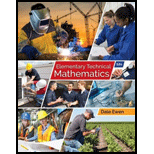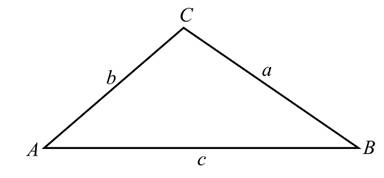# Solve each triangle using the labels as shown in Illustration 1 (round lengths of sides to three significant digits and angles to the east tenth of a degree): ILLUSTRATION 1 a = 38 , 500 mi , b = 67 , 500 mi , c = 47 , 200 mi### Elementary Technical Mathematics

12th Edition
Dale Ewen
Publisher: Cengage Learning
ISBN: 9781337630580

#### Solutions

Chapter
Section### Elementary Technical Mathematics

12th Edition
Dale Ewen
Publisher: Cengage Learning
ISBN: 9781337630580
Chapter 14.5, Problem 5E
Textbook Problem
1 views

## Solve each triangle using the labels as shown in Illustration 1 (round lengths of sides to three significant digits and angles to the east tenth of a degree):ILLUSTRATION 1 a = 38 , 500  mi , b = 67 , 500  mi ,   c = 47 , 200  mi

To determine

To calculate: The round lengths of sides to three significant digits and angles to the nearest tenth

Of a degree for the provided triangle if a=38500mi,b=67500mi and c=47200mi.### Explanation of Solution

Given Information:

The provided triangle is shown below:

Where a, b and c are sides. A, B and C are the values of angle.

Here,

a=38500mi,b=67500mi and c=47200mi

Formula used:

Law of cosines:

a2=b2+c22bccosA

Law of sine:

asinA=bsinB=csinC

Where a, b and c are sides. A, B and C are the values of angle.

Multiplication property of equality:

A=B and AC=BC Are equivalent where C0.

Calculation:

Consider the provided triangle diagram with provided value,

a=38500mi,b=67500mi and c=47200mi

Use law of cosines a2=b2+c22bccosA to find angle A.

Now substitute the value a=38500mi,b=67500mi and c=47200mi in a2=b2+c22bccosA

Therefore,

(38500)2=(67500)2+(47200)22(67500)(47200)cosA1482250000=4556250000+22278400006372000000cosA14822500006784090000=6372000000cosA5301840000=6372000000cosA

Further simplify,

cosA=53018400006372000000cosA=0

### Still sussing out bartleby?

Check out a sample textbook solution.

See a sample solution

#### The Solution to Your Study Problems

Bartleby provides explanations to thousands of textbook problems written by our experts, many with advanced degrees!

Get Started

Find more solutions based on key concepts
In problems 45-62, perform the indicated operations and simplify. 60.

Mathematical Applications for the Management, Life, and Social Sciences

In Exercises 7378, determine whether the statement is true or false. If it is true, explain why it is true. If ...

Applied Calculus for the Managerial, Life, and Social Sciences: A Brief Approach

Draw a polygon for the distribution of scores shown in the following table. X f 6 2 5 5 4 3 3 2 2 1

Essentials of Statistics for The Behavioral Sciences (MindTap Course List)

Convert the expressions in Exercises 31-36 to positive exponent form. 13x4+0.1x23

Finite Mathematics and Applied Calculus (MindTap Course List)

What is the general purpose of a manipulation check?

Research Methods for the Behavioral Sciences (MindTap Course List)

True or False: a × b is a vector in the plane formed by vectors a and b.

Study Guide for Stewart's Multivariable Calculus, 8th

The x-coordinate of the center of mass of the region bounded by , x = 1, x = 2, y = 0 is: ln 2 1 2 ln 2

Study Guide for Stewart's Single Variable Calculus: Early Transcendentals, 8th